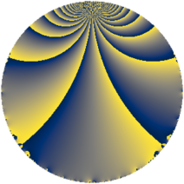# Properties

 Label 56.2.mLevel $56$ Weight $2$ Character orbit 56.m Rep. character $\chi_{56}(3,\cdot)$ Character field $\Q(\zeta_{6})$ Dimension $12$ Newform subspaces $1$ Sturm bound $16$ Trace bound $0$

# Related objects

## Defining parameters

 Level: $$N$$ $$=$$ $$56 = 2^{3} \cdot 7$$ Weight: $$k$$ $$=$$ $$2$$ Character orbit: $$[\chi]$$ $$=$$ 56.m (of order $$6$$ and degree $$2$$) Character conductor: $$\operatorname{cond}(\chi)$$ $$=$$ $$56$$ Character field: $$\Q(\zeta_{6})$$ Newform subspaces: $$1$$ Sturm bound: $$16$$ Trace bound: $$0$$

## Dimensions

The following table gives the dimensions of various subspaces of $$M_{2}(56, [\chi])$$.

Total New Old
Modular forms 20 20 0
Cusp forms 12 12 0
Eisenstein series 8 8 0

## Trace form

 $$12q - 6q^{3} - 12q^{8} + O(q^{10})$$ $$12q - 6q^{3} - 12q^{8} + 6q^{10} - 6q^{11} - 18q^{12} + 6q^{14} - 6q^{17} + 6q^{18} - 6q^{19} + 24q^{22} + 6q^{24} + 6q^{26} + 6q^{28} - 12q^{30} - 6q^{33} + 18q^{35} + 48q^{36} - 24q^{38} + 42q^{40} - 30q^{42} + 6q^{44} - 18q^{46} - 12q^{49} - 48q^{50} + 6q^{51} - 24q^{52} - 36q^{54} - 36q^{57} + 18q^{58} + 42q^{59} - 6q^{60} - 72q^{64} - 12q^{65} + 12q^{66} + 30q^{67} - 36q^{68} + 30q^{70} + 18q^{73} + 12q^{74} + 24q^{75} + 60q^{78} + 36q^{80} + 6q^{81} + 54q^{82} + 12q^{84} + 6q^{88} + 18q^{89} - 72q^{91} + 60q^{92} - 12q^{94} + 60q^{96} - 6q^{98} - 24q^{99} + O(q^{100})$$

## Decomposition of $$S_{2}^{\mathrm{new}}(56, [\chi])$$ into newform subspaces

Label Dim. $$A$$ Field CM Traces $q$-expansion
$$a_2$$ $$a_3$$ $$a_5$$ $$a_7$$
56.2.m.a $$12$$ $$0.447$$ 12.0.$$\cdots$$.2 None $$0$$ $$-6$$ $$0$$ $$0$$ $$q+(\beta _{1}-\beta _{8})q^{2}+(-1-\beta _{10})q^{3}+(\beta _{2}+\cdots)q^{4}+\cdots$$